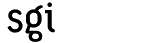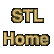# search_nCategory: algorithms Component type: function

### Prototype

Search_n is an overloaded name; there are actually two search_n functions.
```template <class ForwardIterator, class Integer, class T>
ForwardIterator search_n(ForwardIterator first, ForwardIterator last,
Integer count, const T& value);

template <class ForwardIterator, class Integer,
class T, class BinaryPredicate>
ForwardIterator search_n(ForwardIterator first, ForwardIterator last,
Integer count, const T& value,
BinaryPredicate binary_pred);
```

### Description

Search_n searches for a subsequence of count consecutive elements in the range [first, last), all of which are equal to value.  It returns an iterator pointing to the beginning of that subsequence, or else last if no such subsequence exists. The two versions of search_n differ in how they determine whether two elements are the same: the first uses operator==, and the second uses the user-supplied function object binary_pred.

The first version of search returns the first iterator i in the range [first, last - count)  such that, for every iterator j in the range [i, i + count), *j == value. The second version returns the first iterator i in the range [first, last - count) such that, for every iterator j in the range [i, i + count), binary_pred(*j, value) is true.

### Definition

Defined in the standard header algorithm, and in the nonstandard backward-compatibility header algo.h.

### Requirements on types

For the first version:
• ForwardIterator is a model of Forward Iterator.
• Integer is an integral type.
• T is a model of EqualityComparable.
• ForwardIterator's value type is a model of EqualityComparable.
• Objects of ForwardIterator's value type can be compared for equality with Objects of type T.
For the first version:
• ForwardIterator is a model of Forward Iterator.
• Integer is an integral type.
• T is a model of EqualityComparable.
• BinaryPredicate is a model of Binary Predicate.
• ForwardIterator's value type is convertible to BinaryPredicate's first argument type.
• T is convertible to BinaryPredicate's second argument type.

### Preconditions

• [first, last) is a valid range.
• count is non-negative .

### Complexity

Linear. Search_n performs at most last - first comparisons.

(The C++ standard permits the complexity to be O(n (last - first)), but this is unnecessarily lax. There is no reason for search_n to examine any element more than once.)

### Example

```bool eq_nosign(int x, int y) { return abs(x) == abs(y); }

void lookup(int* first, int* last, size_t count, int val) {
cout << "Searching for a sequence of "
<< count
<< " '" << val << "'"
<< (count != 1 ? "s: " : ":  ");
int* result = search_n(first, last, count, val);
if (result == last)
else
cout << "Index = " << result - first << endl;
}

void lookup_nosign(int* first, int* last, size_t count, int val) {
cout << "Searching for a (sign-insensitive) sequence of "
<< count
<< " '" << val << "'"
<< (count != 1 ? "s: " : ":  ");
int* result = search_n(first, last, count, val, eq_nosign);
if (result == last)
else
cout << "Index = " << result - first << endl;
}

int main() {
const int N = 10;
int A[N] = {1, 2, 1, 1, 3, -3, 1, 1, 1, 1};

lookup(A, A+N, 1, 4);
lookup(A, A+N, 0, 4);
lookup(A, A+N, 1, 1);
lookup(A, A+N, 2, 1);
lookup(A, A+N, 3, 1);
lookup(A, A+N, 4, 1);

lookup(A, A+N, 1, 3);
lookup(A, A+N, 2, 3);
lookup_nosign(A, A+N, 1, 3);
lookup_nosign(A, A+N, 2, 3);
}
```

The output is

```Searching for a sequence of 1 '4':  Not found
Searching for a sequence of 0 '4's: Index = 0
Searching for a sequence of 1 '1':  Index = 0
Searching for a sequence of 2 '1's: Index = 2
Searching for a sequence of 3 '1's: Index = 6
Searching for a sequence of 4 '1's: Index = 6
Searching for a sequence of 1 '3':  Index = 4
Searching for a (sign-insensitive) sequence of 1 '3':  Index = 4
Searching for a (sign-insensitive) sequence of 2 '3's: Index = 4
```

### Notes

 Note that count is permitted to be zero: a subsequence of zero elements is well defined. If you call search_n with count equal to zero, then the search will always succeed: no matter what value is, every range contains a subrange of zero consecutive elements that are equal to value. When search_n is called with count equal to zero, the return value is always first.

 The reason that this range is [first, last - count), rather than just [first, last), is that we are looking for a subsequence whose length is count; an iterator i can't be the beginning of such a subsequence unless last - count is greater than or equal to count. Note the implication of this: you may call search_n with arguments such that last - first is less than count, but such a search will always fail.